# 一、算法思想

## 二、算法具体实现（三种实现）

1、前后指针法(重点介绍)

①将数组最左的元素记为key，创建指针i和j，i指向数组最左边的元素，j指向i的下一位。

②比较array[j]和key：如果array[j]>key,j后移一位，i不动。若array[j]<key，交换i+1和j的值，ij同时后移一位。

③当j走到数组的末尾时，先执行②操作，再交换array[i]和key。

④对两边进行上述操作的递归操作。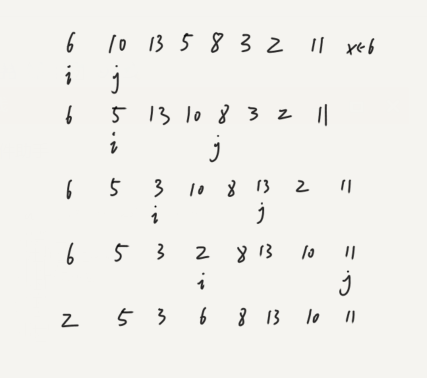j走到5时,5<6，交换5和i+1，即交换5和10，ij同时后移一位。

j走到3时,3<6，交换3和i+1，即交换3和13，ij同时后移一位。

j走到2时,2<6，交换2和i+1，即交换2和10，ij同时后移一位。

j走到11时,11>6，不用交换，此时j到达数组末尾，交换key和i，即交换6和2。

2、左右指针法(非降序)

之所以叫左右指针，是因为初始的两指针是在数据的两端，看作左右，与前后指针法不同的是两指针是往中间移动的。

①将最右的元素定为key，左指针最左的元素，右指针指向最右的元素。

②右指针向左走，如果遇到了比key小的数，右指针停下，接着左指针往右走，遇到比key大的数时停下。交换左右指针的值，两指针再向中间移一位。

③检查两指针是否重合，不重合时进行②操作，重合时将key的值与重合位置的值交换。

④对两边进行上述操作的递归操作。

（放图说明）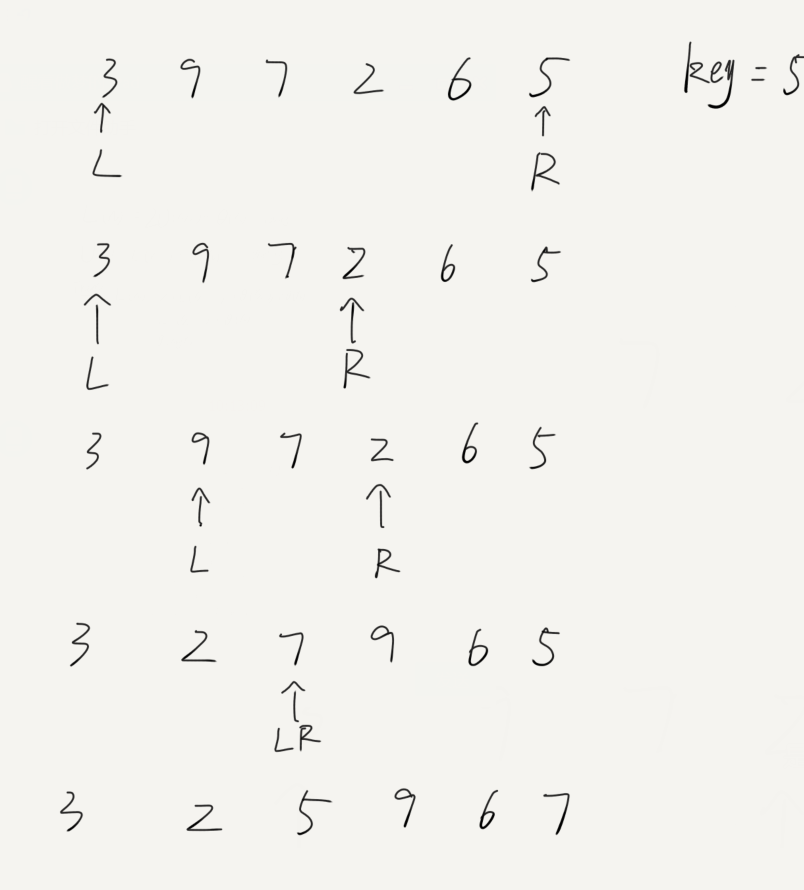3、填坑法（非降序）

填坑法的坑只有一个，是由指针指向且不断变化的，有些类似于左右指针法，具体不同会在下面分析。

①将数组最右的端定为坑，并记下坑值为key（一趟排序只记这一次），左指针最左的元素，右指针指向最右的元素。

②左指针向右移动，当遇到比坑值大的数时停下，将这个数填入坑中，将左指针此时的位置设为坑。右指针向左移，当遇到比key小的数时，将这个数填入坑中，将右指针此时的位置设为坑。

③判断两指针是否重合，若不重合执行②操作，重合就将初始的key值填入重合的位置。至此一趟排序结束。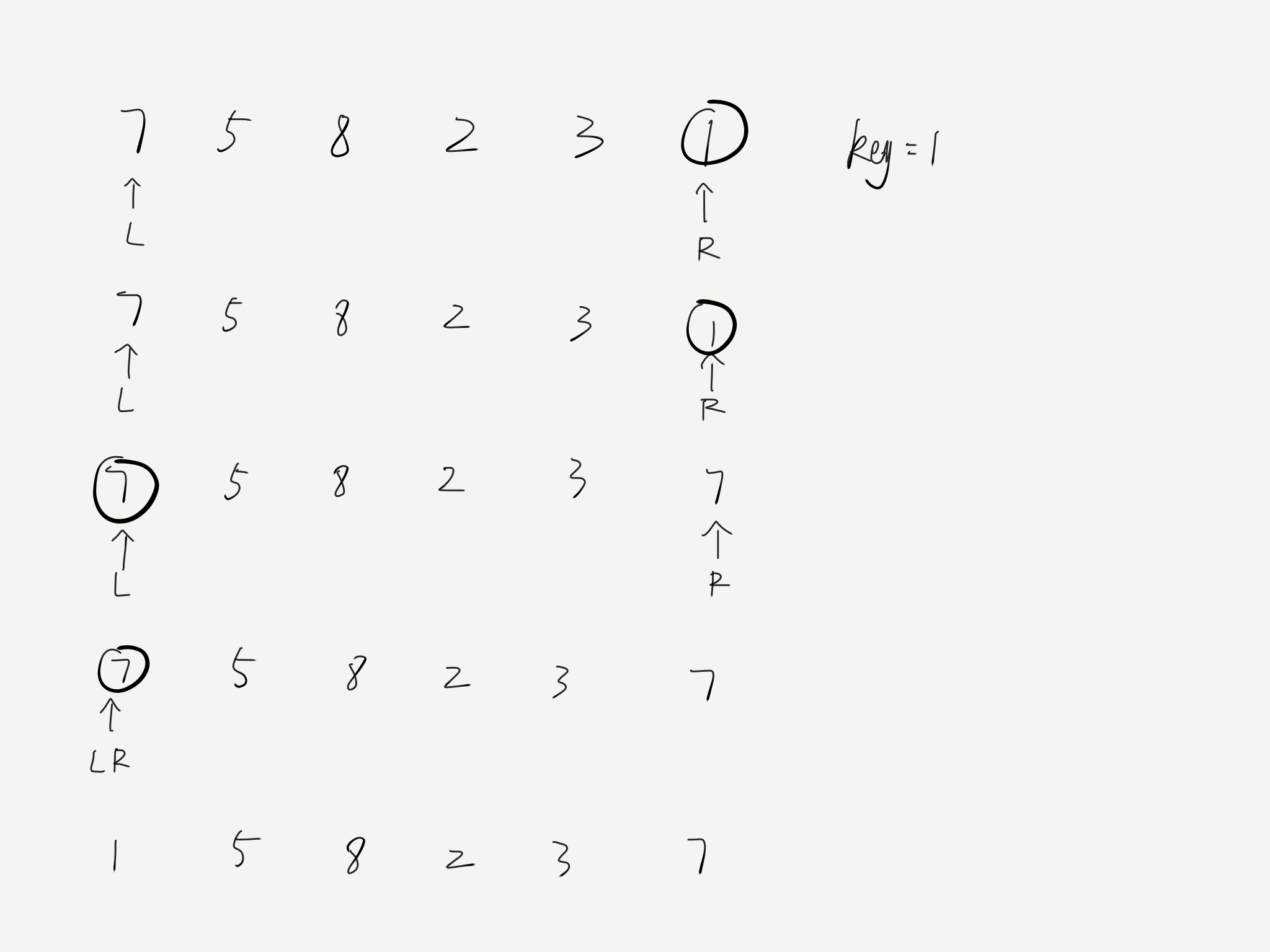（上图中圈圈代表坑）

（这只是一种特殊情况，请读者自己作更多的数组来练习）

### 三、复杂度（要想透彻理解务必看完）

1、最优情况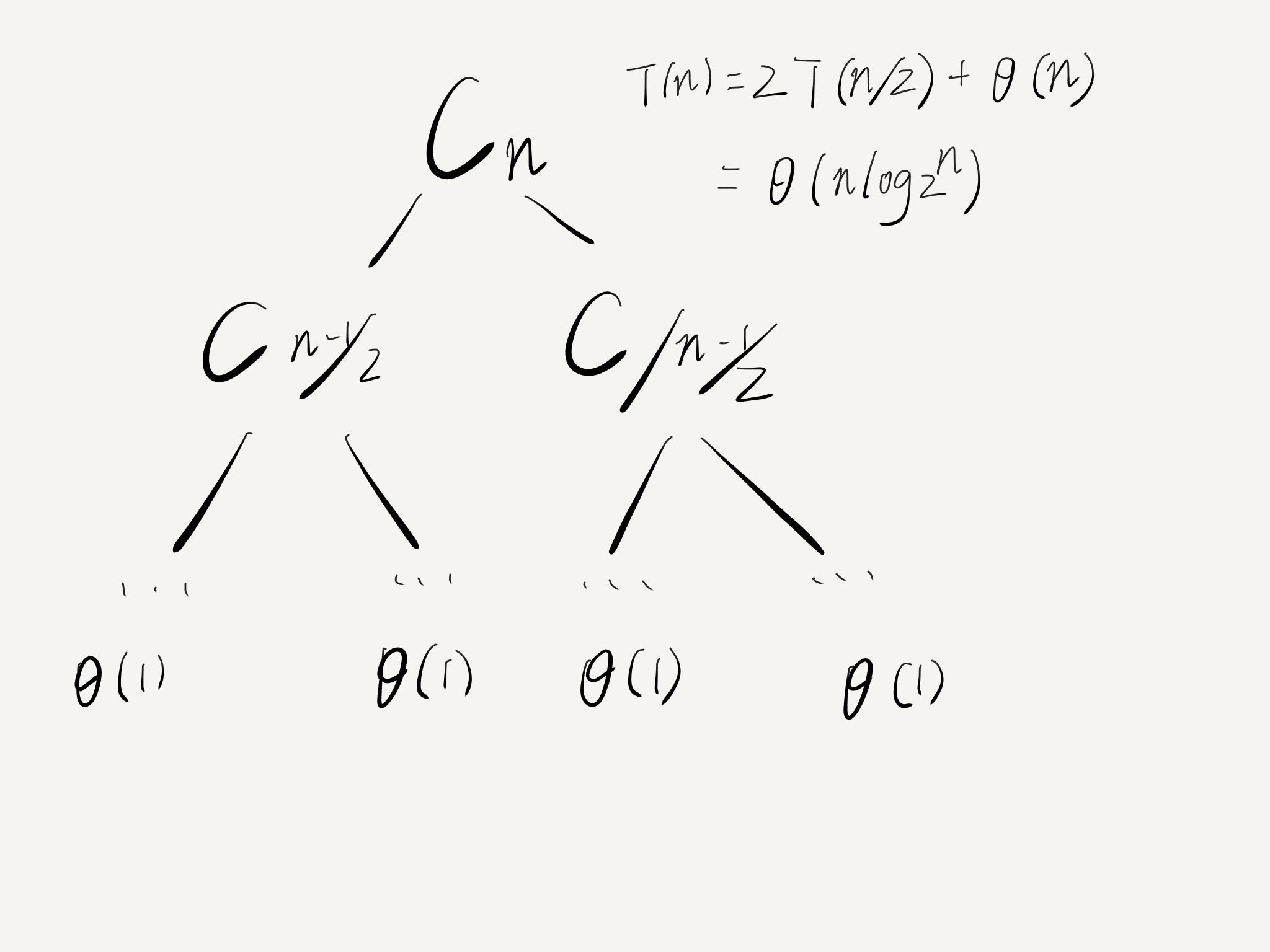2、最坏情况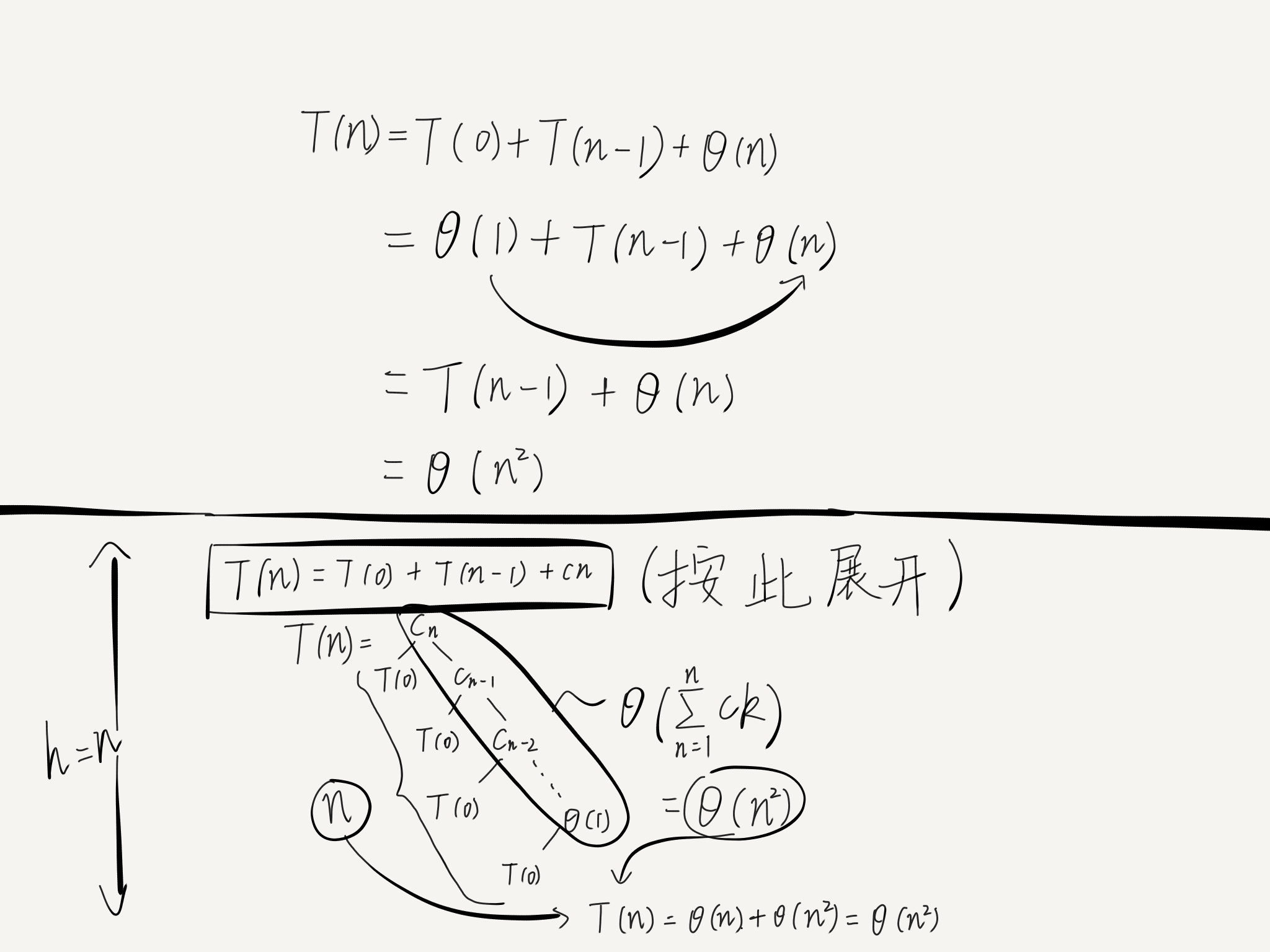3、固定位置排序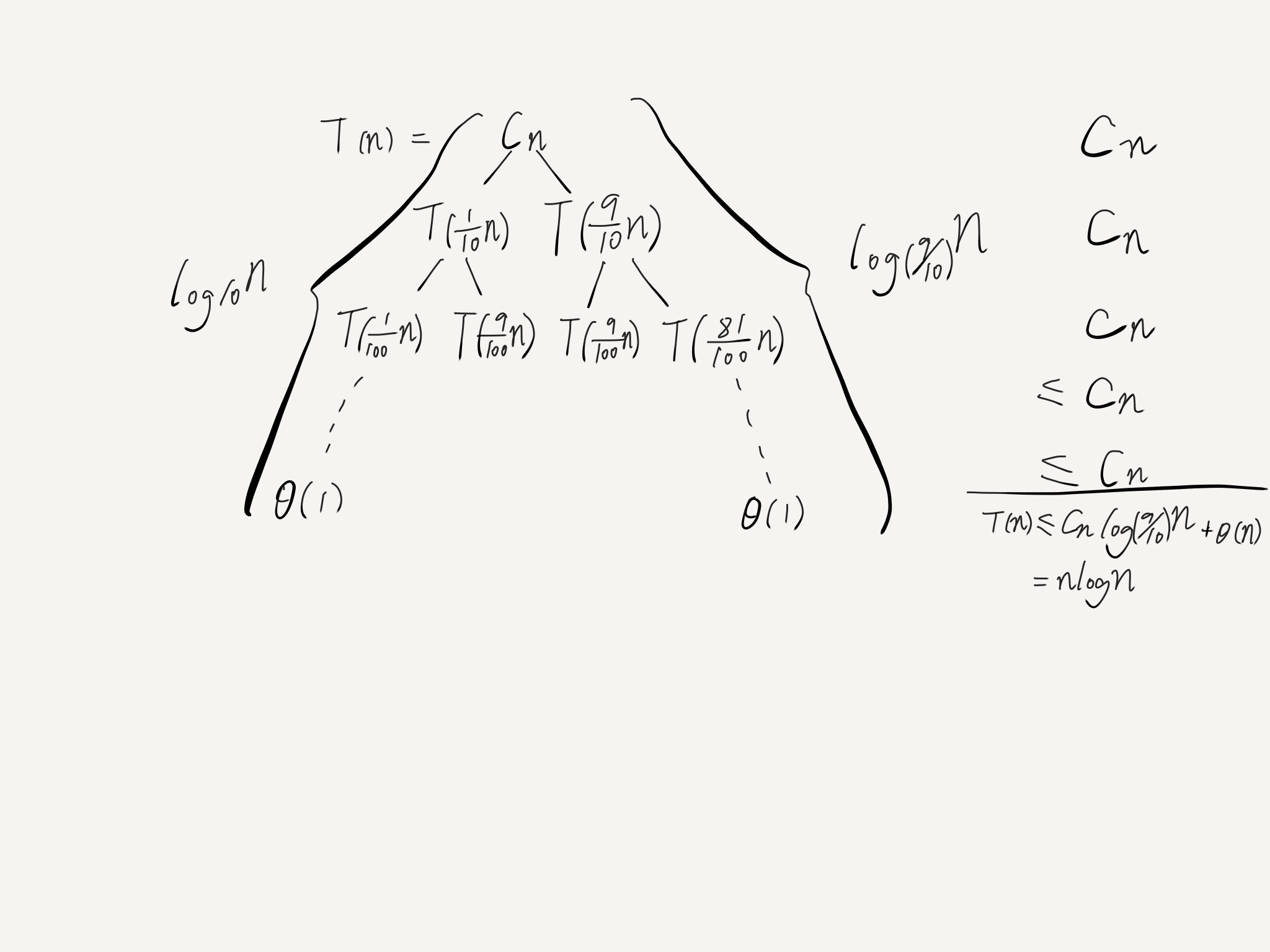4、优劣交替排序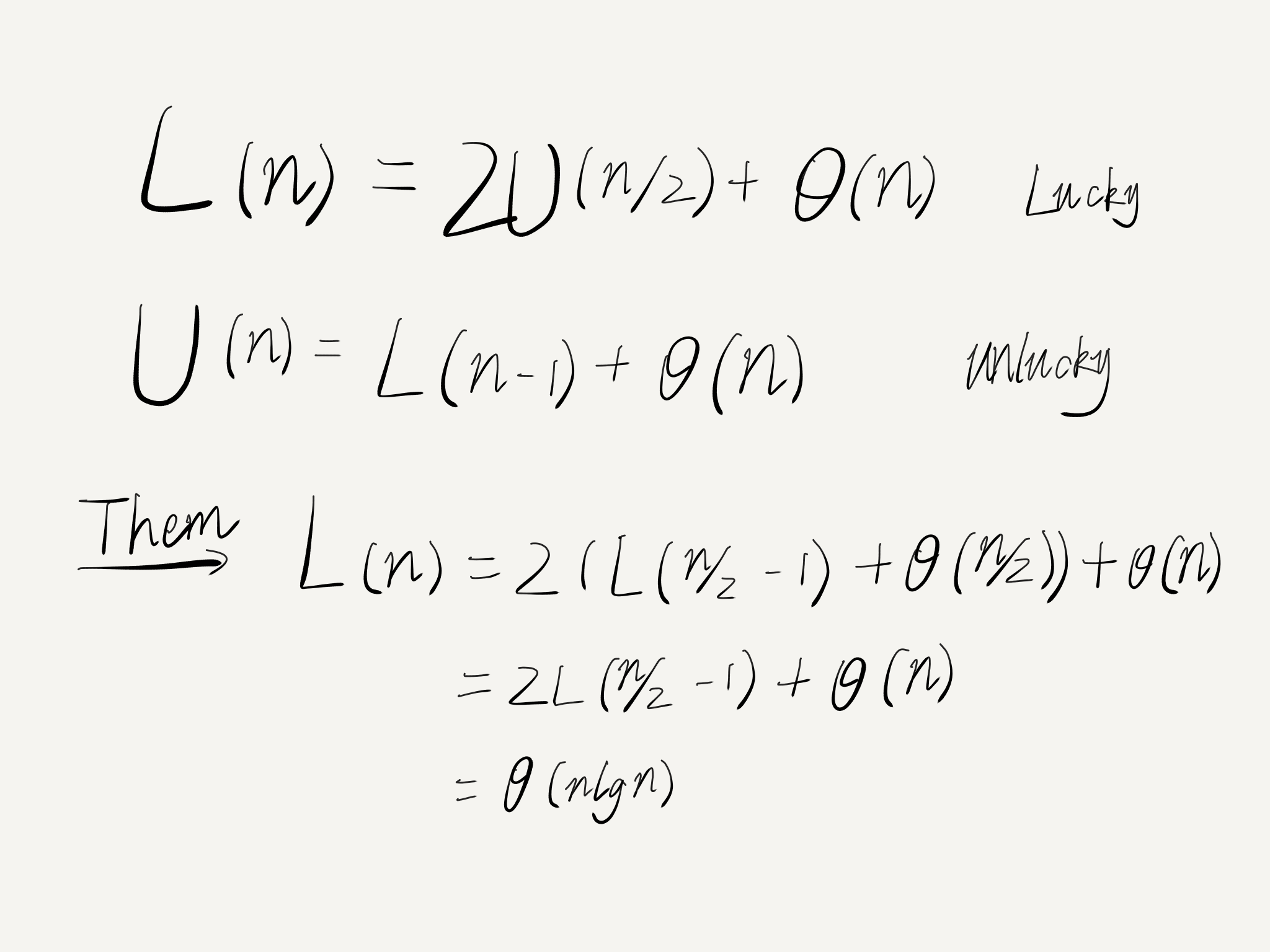them中的式子用了等价代换（只是将n/2带入U（n））

#### 四、算法的优化

1、三数取中法

2、加入插入排序

##### 五、代码展示

1、前后指针法

 1 #include<iostream>
2 using namespace std;
3 #include<assert.h>
4 int GetMidIndex(int *a,int left,int right);
5 int PartSort(int *a,int left,int right);
6 void QuickSort(int *a,int left,int right);
7 void InsertSort(int* a,int n);
8 void printArray(int *a,int n);
9 int main()
10 {
11     int arr[] = { 1, 3, 6, 0, 8, 2, 9, 4, 7, 5 };
12     //int arr[] = { 49, 38, 65, 97, 76, 13, 27, 49 };
13     int n = sizeof(arr) / sizeof(arr);
14     cout << "进行快速排序后：";
15     QuickSort(arr, 0, n - 1);
16     printArray(arr, n);
17     system("pause");
18     return 0;
19 }
20
21 int GetMidIndex(int *a,int left,int right)
22 {//三数取中法
24     int mid=left+(right-left)/2;
25     if(a[left]>=a[mid]){
26         max=a[left];
28     }
29     else{
30         max=a[mid];
32     }
33     if(a[right]>max){
34         max=a[right];
36     }
37 }
38
39 int PartSort(int *a,int left,int right)
40 {//前后指针法的实现
41     int mid=GetMidIndex(a,left,right);
42     swap(a[mid],a[left]);
43     int key=a[left];
44     int j=left+1;
45     int i=left;
46     while(j<=right&&i<j){
47         if(a[j]<key&&++i!=j){
48             swap(a[i],a[j]);
49         }
50         j++;//如果最后一个也比key小，j就会超出数组
51     }
52     swap(a[left],a[i]);//交换key值和array[i]
53     return i;
54 }
55
56 void QuickSort(int *a,int left,int right)
57 {//快排主要思想（分治法
58     if(left>=right){
59         return;
60     }
61     if((right-left)<5){
62         InsertSort(a,right-left+1);
63     }
64     int div=PartSort(a,left,right);
65     QuickSort(a,left,div-1);
66     QuickSort(a,div+1,right);
67 }
68
69 void InsertSort(int* a,int n)//当数组规模较小或者存在多个局部有序的子数组时，算法的执行速度会更快
70 {//插入排序 每次将一个元素插入以排好的序列
71     for(int i=1;i<n;i++){
72         for(int j=i;j>0&&a[j]<a[j-1];j--){
73             swap(a[j],a[j-1]);
74         }
75     }
76 }
77 void printArray(int *a,int n)
78 {
79     for(int i=0;i<n;i++){
80         printf("%d ",a[i]);
81     }
82 }

2、左右指针法

#include<iostream>
using namespace std;
#include<assert.h>

int PartSort(int* array,int left,int right);
void QuickSort(int* array,int left,int right);
int GetMidIndex(int* a,int left,int right);
void InsertSort(int* a,int n);
void PrintArray(int* a,int n);
int main()//快速排序（填坑法）
{
int arr[]={1,4,2,7,8,5,3,9,0,6};
int n=sizeof(arr)/sizeof(arr);
QuickSort(arr,0,n-1);
PrintArray(arr,n);
system("pause");
return 0;
}

int PartSort(int* array,int left,int right)
{
int mid=GetMidIndex(array,left,right);
swap(array[mid],array[right]);
int key=array[right];
while(left<right){//除第一次外，left和right都是从坑开始移动
while(left<right&&array[left]<=key){
++left;
}
array[right]=array[left];
while(left<right&&array[right]>=key){
--right;
}
array[left]=array[right];
}
array[right]=key;
return right;
}

void QuickSort(int* array,int left,int right)
{//快排核心思想（分治法
if(left>=right){
return;
}
if(right-left<=5){
//printf("insert!");
InsertSort(array,right-left+1);
}
int div=PartSort(array,left,right);
QuickSort(array,left,div-1);
QuickSort(array,div+1,right);
}

void InsertSort(int* a,int n)//当数组规模较小或者存在多个局部有序的子数组时，算法的执行速度会更快
{//插入排序 每次将一个元素插入以排好的序列
for(int i=1;i<n;i++){
for(int j=i;j>0&&a[j]<a[j-1];j--){
swap(a[j],a[j-1]);
}
}
}

void PrintArray(int* a,int n)
{
for(int i=0;i<n;i++){
printf("%d ",a[i]);
}
}

int GetMidIndex(int *a,int left,int right)
{//三数取中法（序列是正序或者逆序时，每次选到的枢轴都是没有起到划分的作用。快排的效率会极速退化。
assert(a);
int mid=left+(right-left)/2;
if(a[left]<=a[right]){
if(a[mid]<a[left]){
return left;
}
else if(a[mid]>a[right]){
return right;
}
else{
return mid;
}
}
else{
if(a[mid]<a[right]){
return right;
}
else if(a[mid]>a[left]){
return left;
}
else{
return mid;
}
}
}

3、填坑法

#include<iostream>
using namespace std;
#include<assert.h>

int PartSort(int* array,int left,int right);
void QuickSort(int* array,int left,int right);
int GetMidIndex(int* a,int left,int right);
void PrintArray(int* a,int n);
int main()//快速排序（填坑法）
{
int arr[]={1,4,2,7,8,5,3,9,0,6};
int n=sizeof(arr)/sizeof(arr);
QuickSort(arr,0,n-1);
PrintArray(arr,n);
system("pause");
return 0;
}

int PartSort(int* array,int left,int right)
{
int mid=GetMidIndex(array,left,right);
swap(array[mid],array[right]);
int key=array[right];
while(left<right){//除第一次外，left和right都是从坑开始移动
while(left<right&&array[left]<=key){
++left;
}
array[right]=array[left];
while(left<right&&array[right]>=key){
--right;
}
array[left]=array[right];
}
array[right]=key;
return right;
}

void QuickSort(int* array,int left,int right)
{
if(left>=right){
return;
}
int div=PartSort(array,left,right);
QuickSort(array,left,div-1);
QuickSort(array,div+1,right);
}

void PrintArray(int* a,int n)
{
for(int i=0;i<n;i++){
printf("%d ",a[i]);
}
}

int GetMidIndex(int *a,int left,int right)
{//三数取中法（序列是正序或者逆序时，每次选到的枢轴都是没有起到划分的作用。快排的效率会极速退化。
assert(a);
int mid=left+(right-left)/2;
if(a[left]<=a[right]){
if(a[mid]<a[left]){
return left;
}
else if(a[mid]>a[right]){
return right;
}
else{
return mid;
}
}
else{
if(a[mid]<a[right]){
return right;
}
else if(a[mid]>a[left]){
return left;
}
else{
return mid;
}
}
}
posted @ 2018-08-16 11:23 脑热 阅读(...) 评论(...) 编辑 收藏